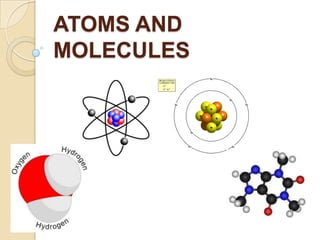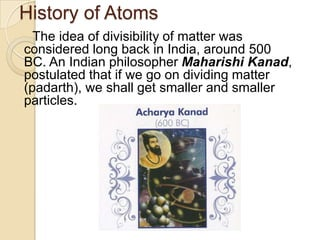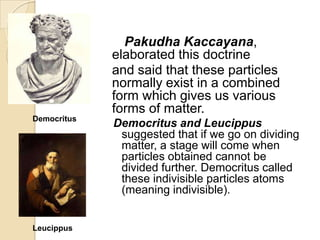Successfully reported this slideshow.

# Atoms and Molecules

27

Share×
1 of 42
1 of 42

# Atoms and Molecules

27

Share

Learn about the basic building blocks of matter i.e. ATOMS. Based on Class 9 Syllabus.

Learn about the basic building blocks of matter i.e. ATOMS. Based on Class 9 Syllabus.

## More Related Content

### Related Books

Free with a 14 day trial from Scribd

See all

### Related Audiobooks

Free with a 14 day trial from Scribd

See all

### Atoms and Molecules

1. 1. ATOMS AND MOLECULES
2. 2. History of Atoms The idea of divisibility of matter was considered long back in India, around 500 BC. An Indian philosopher Maharishi Kanad, postulated that if we go on dividing matter (padarth), we shall get smaller and smaller particles.
3. 3. Pakudha Kaccayana, elaborated this doctrine and said that these particles normally exist in a combined form which gives us various forms of matter. Democritus Leucippus Democritus and Leucippus suggested that if we go on dividing matter, a stage will come when particles obtained cannot be divided further. Democritus called these indivisible particles atoms (meaning indivisible).
4. 4. Father of Modern Chemistry: Antoine L. Lavoiser  Antoine-Laurent de Lavoisier (26 August 1743 – 8 May1794)was a French nobleman and chemist centr al to the 18thcentury Chemical Revolution and a large influence on both the histories of chemistry and biology. Antoine L. Lavoiser laid the foundation of chemical sciences by establishing two important laws of chemical combination.
5. 5. Laws of Chemical Combinations There are two laws of chemical combination.  Law of Conservation of Mass  Law of Constant Proportions
6. 6. Law of Conservation of Mass Antoine L. Lavoisier, a French scientist, established the theory of Law of Conservation of Mass.  The law of conservation of mass states, “Mass can neither be created nor destroyed in a chemical reaction”. 
7. 7. Explanation of the Law:  According to this law mass of an isolated system will remain constant over time. This means when mass is enclosed in a system and none is allowed in or out, its quantity will never change. That is mass will be conserved, and hence this is called Law of Conservation of Mass. This means total mass of products is always equal to the total mass of reactants. As there is no loss of mass of substances, i.e. mass is conserved, that‟s why Lavoisier called this the law of conservation of mass.
8. 8. Reactants and Products: In a chemical reaction  The substances that combine or react are known as reactants.  The new substance/substances formed are called product or products.
9. 9. Example:  When calcium oxide is dissolved in water calcium hydroxide is formed. The reaction involved in this can be written as: In this reaction calcium oxide and water are reactants while calcium hydroxide is product.  In this reaction 74 g of calcium hydroxide is obtained when 56 g of calcium oxide reacts with 18 g of water, which is proved 
10. 10.  Here the total mass of reactants, i.e. calcium oxide and water is equal to 74 g. And the mass of product, i.e. calcium hydroxide is also equal to 74g. This proves that the total mass of reactants is always equal to the total mass of product, which proves the Law of Conservation of Mass.
11. 11. Law of Constant Proportions Law of Constant Proportion states that a chemical compound always contains exactly the same proportion of elements by mass.  This law is also known as Law of definite proportions. Joseph Louis Proust gave this law hence, this law is also known as Proust‟s Law. 
12. 12. Explanation of the law  Compounds are formed by the combination of two or more elements. In a compound the ratio of the atoms or element by mass remains always same irrespective of the source of compound. This means a certain compound always formed by the combination of atoms in same ratio by mass. If the ratio of mass of constituent atoms will be altered the new compound is formed.
13. 13. Examples:      In a water molecule (H20) Hydrogen : Oxygen= 1:8 In Ammonia ( NH3) Nitrogen : Oxygen = 14:3 In Common Salt (NaCl) Sodium: Chlorine= 23: 35.5 In Carbon dioxide (CO2) Carbon: Oxygen = 3:8 In Calcium Oxide (CaO) Calcium: Oxygen= 5:2 In Magnesium Sulphide (MgS) Magnesium: Sulphur = 3:4
14. 14. John Dalton  John Dalton (6 September 1766 – 27 July 1844) was an English chemist, mete orologist and physicist . He is best known for his pioneering work in the development of modern atomic theory, and his research into colour blindness.
15. 15. Dalton’s Atomic Theory  John Dalton, a British Chemists and scientists gave the Atomic Theory in 1808. This theory is popularly known as Dalton‟s Atomic Theory in the honor of John Dalton. He gave the theory on the basis of Laws of Chemical Combination and explains them properly. In his theory he explains about atom.
16. 16. Main postulates of Dalton's atomic theory       Elements are made of extremely small particles called atoms. Atoms of a given element are identical in size, mass, and other properties; Atoms of different elements differ in size, mass, and other properties. Atoms cannot be subdivided, created, or destroyed. Atoms of different elements combine in simple whole-number ratios to form chemical compounds. In chemical reactions, atoms are combined, separated, or rearranged.
17. 17. What is an Atom?  Atom can be defined as the smallest particle of matter that takes part in a chemical reaction. How big are Atoms? Atoms are very small, they are smaller than anything that we can imagine or compare with.
18. 18. Characteristics of atoms:         Atom is the smallest particle of matter. All elements are made of tiny particles called atom. Atoms are very small in size and cannot be seen through naked eyes. Atom does not exist in free-state in nature. But atom takes part in a chemical reaction. The properties of a matter depend upon the characteristics of atoms. Atoms are the building block of an element similar to a brick which combine together to make a building. The size of atoms is indicated by its radius. In ancient time atoms was considered indivisible.
19. 19. Symbols of Atoms of Different Elements Symbols of some elements are formed from the first letter of the name and a letter, appearing later in the name. E.g.: (i) chlorine: Cl, (ii) zinc: Zn etc Some have been taken from the names of elements in Latin, German or Greek. E.g.: s Iron is Fe from ferrum, Sodium is Na from natrium, Potassium is K from kalium
20. 20. Atomic Mass  Mass of atom is called atomic mass. Since, atoms are very small consequently actual mass of an atom is very small. For example the actual mass of one atom of hydrogen is equal to 1.673 x 10-24 g. This is equal to 0.000000000000000000000001673 gram. To deal with such small number is very difficult. Thus for convenience relative atomic mass is used.
21. 21. Existence of Atoms Atoms of most of the elements exist in the form of molecule or ion, since they are most reactive. For example, hydrogen, oxygen, chlorine, etc. However, atoms of some elements, which are non-reactive, exist in free-state in nature. For example helium, neon, argon, etc.  Usually atoms are exist in following two forms 1. In the form of molecules 2. In the form of ions 
22. 22. Molecule  A molecule is a group of two or more atoms that are chemically bonded together, that is, tightly held together by attractive forces.
23. 23. Atomicity    Mono-atomic: When molecule is formed by single atom only, it is called mono-atomic molecule. Generally noble gases form monoatomic molecules. For example: Helium (He), Neon (Ne), Di-atomic: When molecule is formed by the combination of two atoms of it is called diatomic molecule. For example: Hydrogen (H2), Oxygen (O2), Nitrogen (N2), Chlorine (Cl2), etc. Tri-atomic: When molecule is formed by the combination of three atoms it is called tri-atomic molecule. For example: molecule of ozone (O3)
24. 24. Tetra-atomic:- When molecule is formed by the combination of four atoms it is called tetra-atomic molecule. For example: Phosphorous molecule (P4)  Polyatomic:- When molecule is formed by the combination of more than two atoms, it is called polyatomic molecule. For example: Sulphur molecule (S8)  All metals are mono-atomic. All gases are di-atomic.
25. 25. Molecules of Elements The molecules of an element are constituted by the same type of atoms. e.g.: O + O→ O2 (Oxygen Gas) „diatomic‟ O + O + O→ O3 (Ozone) „tri-atomic‟ 4P→ P4 (Phosphorous) „tetraatomic‟ 8S→ S8 (Sulphur) „poly-atomic‟ 
26. 26. Molecules of Compound Atoms of different elements join together in definite proportions to form molecules of compounds. e.g.: Ca + O → CaO (Calcium Oxide) „diatomic‟ 2H + O → H20 (Water) „tri-atomic‟ N + 3H → NH3 (Ammonia) „tetra-atomic‟ 2K + S + 4O → K2SO4 (Potassium sulphate) „polyatomic‟ 
27. 27. Ions Particles carrying positive or negative charges are called ions. Types of ions: 1. Cations: Positively charged ions E.g.: Al3+, Ca2+ 2. Anions: Negatively charged ions E.g.: Cl−, Br−  All metals form cations by loosing electrons. All non-metals form anions by gaining electrons.
28. 28. Valencies of some elements
29. 29. Chemical Formula The chemical formula of a compound is a symbolic representation of its composition. e.g.: H20 (Water), CaO (Calcium Oxide), NH3 (Ammonia) , K2SO4 (Potassium sulphate) etc. 
30. 30. Rules of writing chemical formulae • The valencies or charges on the ion must balance. • When a compound consists of a metal and a non-metal, the name or symbol of the metal is written first. For example:calcium oxide (CaO), sodium chloride (NaCl), iron sulphide (FeS), copper oxide (CuO) etc., where oxygen, chlorine, sulphur are non-metals and are written on the right, whereas calcium, sodium, iron and copper are metals, and are written on the left. • In compounds formed with polyatomic ions, the ion is enclosed in a bracket before writing the number to indicate the ratio.
31. 31. Formulae of Compounds  Hydrogen chloride  Hydrogen Sulphide
32. 32.  Magnesium chloride  Calcium oxide  Ammonium Sulphate
33. 33.  Sodium carbonate  Calcium hydroxide  Sodium Nitrate
34. 34. Mole Concept One mole of any species (atoms, molecules, ions or particles) is that quantity in number having a mass equal to its atomic or molecular mass in grams.  1 mole (of anything) = 6.022×1023 in number Mass of 1 mole of a particular substance is always fixed. 
35. 35. Avogadro‟s Number It was named after the Italian scientist named Amedeo Avagadro.  It is denoted by.  Its value is 6.022×1023  Ao is equal to 1 mole. 
36. 36. Made By: Samyak Jain